### Arrhenius Theory and Reaction Coordinates

The Arrhenius theory and reaction coordinates relate to a particular perspective on chemical kinetics that imagines the path taken by the reactants as they are converted to the products.  This change is not instantaneous and is typically slowed by the fact that in order to convert from reactants to products, the system must first pass through a higher energy state known as the transition state.  The size of the energy barrier controls the temperature dependence of the rate constant.

At any given temperature there is a distribution of energies for the molecules in the system. Some small fraction have enough energy to get over this barrier. The reaction rate then depends on the number of molecules that have sufficient energy. To speed up the rate, one can either raise the temperature or one can or lower the barrier. As a result, essentially all chemical reaction increase in rate as the temperature is increased. This is the idea that is the basis for the Arrhenius Law. However, this has its limits in the real world since maintaining a system at high temperatures requires energy. In addition, as the temperature increase there may be undesired outcomes such as decomposition of some of the reactants or products. From a practical stand point to speed up a reaction you would like to lower the barrier. This is what is done by a "catalyst" for a given reaction.

### Reaction Coordinate

A reaction coordinate is a path that links the reactant molecules and the products molecules.  In many reactions, we can directly envision this coordinate as the length of a particular bond or bonds.  In other cases, the reaction coordinate is used merely to represent some unknown coordinate.  The key is that there are many many potential paths between reactants and products.  The reaction coordinate represents the lowest energy path.

For example, in the reaction of CH3Cl + OH- to form CH3OH and Cl-, the mechanism of this reaction is a single step in which the CH3Cl collides with the OH- and forms the products.  We can envision a reaction coordinate for this reaction which is the lengthening of the C-Cl bond (the one that is breaking) and the shortening of the C-O bond (the one that is forming).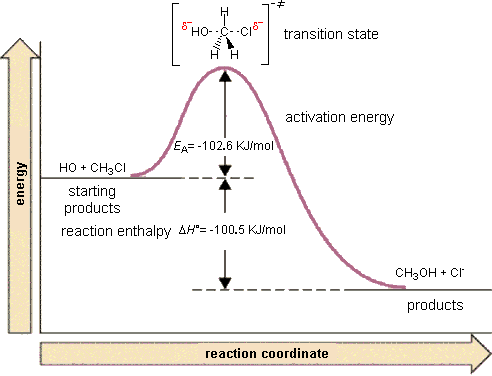Right in the middle of the breaking of the old bond and forming of the new bond, the system exists in what we call a "transition state" that is higher in energy than either the reactants or the products.  Once it gets beyond this state, it proceeds along the reaction coordinate to form the products.

If a chemical mechanism has many steps, then it will have many hills and valleys along the reaction coordinate.  Any minima that exist between the reactants and the products along the reaction coordinate are intermediates.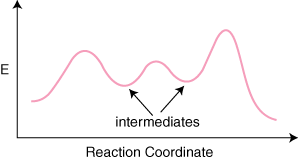The reaction above has three steps (three barriers) and two intermediates.  On the far left of the diagram are the reactant species and on the far right are the product species.

### Transition State

The transition state is the high energy point between two minima along the reaction coordinate.  Each step in a mechanism will have a transition state.  As with the overall mechanism the rate of the reaction may only depend on the highest energy transition state as this will dominate the rate. Experimentally, the transition state is nearly impossible to observed or identify. This is because the molecules exist in this state for essentially zero time.  Therefore, this is not a chemical species we can easily verify in the lab. However, from a theoretical point of view it is a critical concept. The configuration at the transition state is what is determining the rate. The initial and final states of the reaction essentially don't matter. What matters most is the configuration of the transition state. This is the point of maximum energy that the chemical species must pass through during the reaction. It is also important to realize that the reaction path we envision is the minimum energy path between the reactants and the products. There are other potential pathways but these require even more energy (have even higher energy transition states). Therefore the transition state of interest for a given reaction is the highest energy point along the lowest energy path.

### Transition State

The transition state is the higher energy point between two minima along the reaction coordinate.  Each step in a mechanism will have a transition state.  The transition states are often very difficult to identify and during a reaction the molecules exist in this state for essentially zero time.  Therefore, this is not a chemical species we can identify in the lab.  It is more of a theoretical idea for the configurations the chemical species must pass through during the reaction.

### Activation Energy

The height of a barrier along the reaction pathway is the activation energy.  The size of the activation energy depends on which way you approach the barrier as the energy on either side of the barrier could be higher or lower.  Typically, we envision reactions proceeding left to right along the reaction coordinate, so often, the activation energy is only noted for the forward reaction.  The activation energy on the diagram below shows the barrier to be 102.6 kJ mol-1.  Barriers are measured in energy per mole (typically kJ mol-1).### Arrhenius Law and Temperature Dependence

The rate of a reaction depends on the height of the barrier (the activation energy) because only a small fraction of the number of molecules at a given temperature have sufficient energy to get over the barrier.  At a given temperature, there is a distribution of kinetic energies for all the molecules in a sample (this is the Boltzmann distribution).  This molecular kinetic energy can describe things like vibrations of molecules even for samples like solids that might not be "moving".

This distribution has a long tail to high energy.  So there are always a few molecules with sufficient energy to get over the barrier (energies greater than Ea).  As the temperature is raised, the distribution shifts to higher energy and so more molecules have sufficient energy to get over the barrier.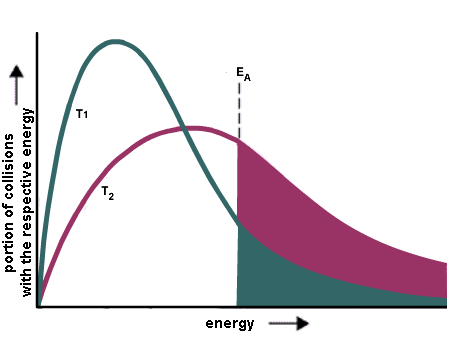This can be quantified using the Boltzmann distribution such that we can write down an expression for the rate constant as a function of temperature

$k = A \exp\left({-E_a \over RT}\right)$

Where k is the rate constant, Ea is the activation energy,  R is the gas constant, A is a constant called the pre-exponential factor, and T is the temperature.  This formula is essentially a comparison between the energy of the molecules (RT) and the energy of the barrier (Ea).  The pre-exponential factor is a constant that is specific to the reaction and it is essentially the ultimate rate of the reaction.  This is the fastest the reaction could ever proceed (if the barrier were zero or the temperature were infinite).

This leads to a very general conclusion.   A barrier exists for most all chemical reactions.  Therefore as the temperature increases, the rate of all reactions increase.  This has little to do with the molecules moving faster and colliding more often.  It has everything to do with the molecules having more energy and making it over the barrier along the reaction coordinate.

Raise the temperature and the reaction will go faster.  Why?  The rate constant is larger at higher temperatures.

The Arrhenius equation is often used to compare the rate constants measured at two different temperatures.  Using two sets of data points, the equation can be rearranged to yield

$\ln \left({k_2 \over k_1} \right) = {-E_a \over R} \left({1 \over T_2} - {1 \over T_1} \right)$

or

$\ln \left({k_2 \over k_1} \right) = {E_a \over R} \left({1 \over T_1} - {1 \over T_2} \right)$

This equation can also be written as

$\ln k = \ln A - {E_a \over RT}$

Therefore if one measures rate constants at many temperatures and constructs a plot of lnk vs 1/T the slope of the plot will be -Ea/R and the intercept will be lnA.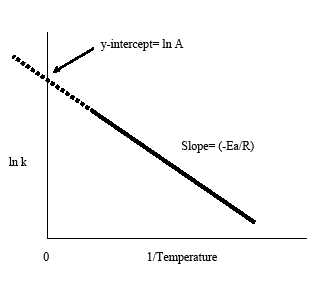Arrhenius Law
Arrhenius Law Example

### Temperature Dependence

This leads to a very general conclusion.   A barrier exists for most all chemical reactions.  Therefore as the temperature increases, the rate of all reactions increase.  This has little to do with the molecules moving faster and colliding more often.  It has everything to do with the molecules having more energy and making it over the barrier along the reaction coordinate.

Raise the temperature and the reaction will go faster.  Why?  The rate constant is larger at higher temperatures.

### Reaction Coordinates and Mechanism

We can relate our reaction coordinate picture to the chemical mechanism.

For example, if we have a mechanism that has three steps, we would expect this reaction to have three barriers.  We would also expect the reaction to have two intermediates.

For example, the following picture illustrates a mechanism that occurs in three steps and forms two intermediates at points B and C (A is reactants and D is products).  Note: Each letter does not literally have to be a single chemical species, instead point B is all the intermediate chemical species that exist after the first step.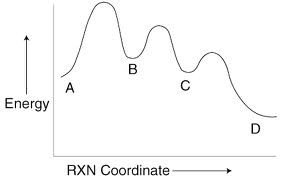Reaction mechanisms also have "fast" and "slow" steps.  We can always identify the "rate limiting step" as it should have the largest barrier.  In the picture above the first step would be the rate limiting step since it has the highest barrier.

Writing out this mechanism we would have

Step 1    A  →  B   slow
Step 2    B  →  C   fast
Step 3    C →  D   fast